# Three equal forces applied to a rectangle, find net torque direction?

• paulimerci

#### paulimerci

Homework Statement
Attached below.
Relevant Equations
T = F.d
This is how I interpreted the problem,
a) The net torque about point A is zero. This is because the forces F1 and F2 are equal and opposite, and they act at the same distance from point A. Therefore, they produce torques that cancel each other out..
The force F3 doesn’t does not produce any torque because it acts along the line of action of F1 and F2 and it passes through the COM.
b) The net torque about point B is counterclockwise because the forces F1 and F2 produce torques that are both CCW, while the force F3 doesn’t produce a torque.
c) The net torque at point c is zero, because it's moment is zero.

I'm not sure with the answers I gave, please point out what mistakes I did. Thank you!

#### Attachments

•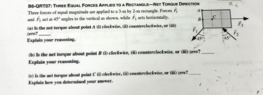Screenshot 2023-03-27 at 11.17.33 AM.png
49.8 KB · Views: 22

Part (a)
F1 and F2 are not equal and opposite. Their sum is not zero. Also, when calculating torques be sure you multiply the magnitude of the force by the lever arm. What are the lever arms of F1 and F2?

Part (b)
You are correct that F3 produces no torque. Are you sure that F1 and F2 both produce ccw torques about point B? Check again.

Part (c) is correct.

•paulimerci
Part (a)
F1 and F2 are not equal and opposite. Their sum is not zero. Also, when calculating torques be sure you multiply the magnitude of the force by the lever arm. What are the lever arms of F1 and F2?

Part (b)
You are correct that F3 produces no torque. Are you sure that F1 and F2 both produce ccw torques about point B? Check again.

Part (c) is correct.
Thank you, for Part a) I know how to find a lever arm for forces but in this case I don't know how to do it. If I take c as the axis of rotation, the line of action for force F1 is crossing the point c in that case it has no moment. Which point should I take, should I take A the axis of rotation?

Thank you, for Part a) I know how to find a lever arm for forces but in this case I don't know how to do it. If I take c as the axis of rotation, the line of action for force F1 is crossing the point c in that case it has no moment. Which point should I take, should I take A the axis of rotation?
Yes, for part (a) you need to take point A as the axis of rotation because that is what the problem is asking you to do.

Yes, for part (a) you need to take point A as the axis of rotation because that is what the problem is asking you to do.
Thank you, If A is the axis of rotation, then F1 produces a CCW torque, and F2 is parallel to the moment arm, so it doesn’t produce any torque. where F3 will exert a CW torque. Correct?

The only statement that is correct is that F2 produces no torque. Also, it is not enough to say what the direction of the torques for F1 and F3 are. If they are in opposite directions, you need to find which is larger which means you need to find their moment arms in order to calculate the net torque.

•paulimerci
The only statement that is correct is that F2 produces no torque. Also, it is not enough to say what the direction of the torques for F1 and F3 are. If they are in opposite directions, you need to find which is larger which means you need to find their moment arms in order to calculate the net torque.
The torque of F3 must be greater than F1 because the lever arm from point A is perpendicular to F1. Whereas the lever arm makes obtuse to the force F3.

Hi,

Are you aware you can shift a force along its line of action ? If you shift ##F_3## to point ##B## and ##F_1## to ##C##, which of the two has a longer 'arm'?

##\ ##

•paulimerci and Lnewqban
Hi,

Are you aware you can shift a force along its line of action ? If you shift ##F_3## to point ##B## and ##F_1## to ##C##, which of the two has a longer 'arm'?

##\ ##
I understand what you say but am unable to verbalize it. I think F1 has a longer arm.

I understand what you say but am unable to verbalize it. I think F1 has a longer arm.
Since F1 has a longer arm than F3, it makes more torque than F3. F1 is CW, and F3 is CCW. Right?

Since F1 has a longer arm than F3, it makes more torque than F3. F1 is CW, and F3 is CCW. Right?
Part b): The forces F1 and F2 produce equal and opposite torques about point c, while the force F3 doesn't exert any torque. Hence, the net torque at B is zero. correct?

Part b): The forces F1 and F2 produce equal and opposite torques about point c, while the force F3 doesn't exert any torque. Hence, the net torque at B is zero. correct?
Yes.
Note that, because A is in the line of action of F2, you can instead treat F2 as being applied at A. This produces a symmetry.

•paulimerci and BvU
Can't resist... does this help ?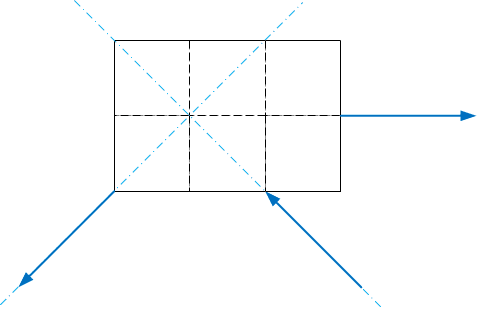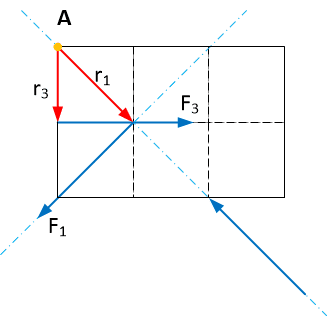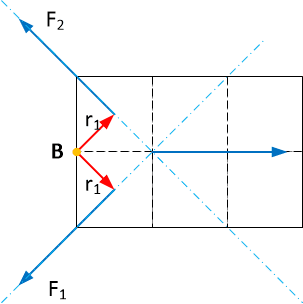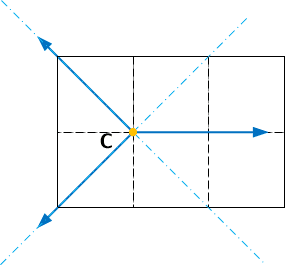spot the mistake: top red arrow in B is r2 , not r1

Last edited:
•paulimerci
Yes.
Note that, because A is in the line of action of F2, you can instead treat F2 as being applied at A. This produces a symmetry.
Thank you!

Can't resist... does this help ?
For part A) The moment arm r1 is greater than r3. Therefore, the F1 exerts more torque than the F3. F1 is a CW torque, and F3 is CCW. The answer for part a is CW. Right?

Right.

•paulimerci and BvU
If you still have to ask, we haven't succeeded in explaining adequately ... ?

On the other hand, if you were in error, there would have been a 'gentle' nudge in the right direction•kuruman
If you still have to ask, we haven't succeeded in explaining adequately ... ?

On the other hand, if you were in error, there would have been a 'gentle' nudge in the right directionSorry for prenudging you.

•SammyS
If you still have to ask, we haven't succeeded in explaining adequately ... ?

On the other hand, if you were in error, there would have been a 'gentle' nudge in the right directionIs there any explanation that I need to provide?

Is there any explanation that I need to provide?
Even though you don't have numbers, you know that the forces are equal in magnitude. Call that ##F##. As far a distances are concerned, call the side of each square ##L##. Then each torque will be some fraction or multiple of ##FL##. Add the two ##FL##s together and see what you get. Equations provide the most convincing explanations.

Is there any explanation that I need to provide?
Just a different approach:
I would vectorially add the three forces.
The resultant force should be applied at the common point C.

Imaginarily locating the pivot point at A for a), B for b) and C for c), would allow me to intuitively see how the resultant force would make the rectangle rotate.

Note that there is a non-stable balance for the case that B is the pivot point, eventually causing a rotation (CCW or CW) until reaching a stable balance.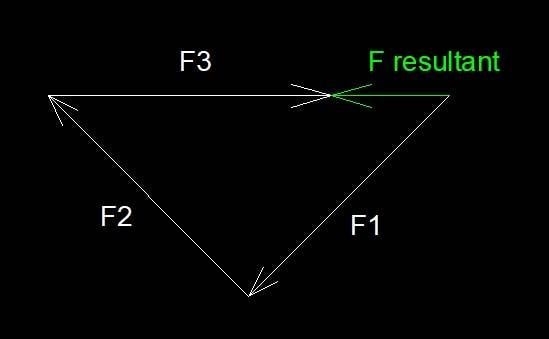Last edited:
•paulimerci
Thank you,
##F1 = F2 = F3 =F ##, ## L2=L3=L1=L4=L##
For part a)
$$\sum \tau_A = -F L\sqrt 2 + FL$$
For part b)
$$\sum\tau_B = F \frac {L \sqrt 2}{2} - F \frac {L \sqrt 2}{2}$$
For part c)
$$\sum\tau_C = 0$$
Do the equations look convincing?

Just a different approach:
I would vectorially add the three forces.
The resultant force should be applied at the common point C.

Imaginarily locating the pivot point at A for a), B for b) and C for c), would allow me to intuitively see how the resultant force would make the rectangle rotate.

Note that there is a non-stable balance for the case that B is the pivot point, eventually causing a rotation (CCW or CW) until reaching a stable balance.

View attachment 324181
It looks like a good strategy! Thanks for sharing.

•Lnewqban
Thank you,
##F1 = F2 = F3 =F ##, ## L2=L3=L1=L4=L##
For part a)
$$\sum \tau_A = -F L\sqrt 2 + FL$$
For part b)
$$\sum\tau_B = F \frac {L \sqrt 2}{2} - F \frac {L \sqrt 2}{2}$$
For part c)
$$\sum\tau_C = 0$$
Do the equations look convincing?
Does the equation look correct? Mistakes?

Thank you,
##F1 = F2 = F3 =F ##, ## L2=L3=L1=L4=L##
For part a)
$$\sum \tau_A = -F L\sqrt 2 + FL$$
For part b)
$$\sum\tau_B = F \frac {L \sqrt 2}{2} - F \frac {L \sqrt 2}{2}$$
For part c)
$$\sum\tau_C = 0$$
Do the equations look convincing?
Yes, but you have not finished part b.

Yes, but you have not finished part b.
Thank you; I think I've included all the forces in the equation for Part B. What is missing?

Thank you; I think I've included all the forces in the equation for Part B. What is missing?

Part b): The force F3 acting along the point B where its moment is zero is why I didn't include it in my equation.
$$\sum\tau_B = F \frac {L \sqrt 2}{2} - F \frac {L \sqrt 2}{2} + F (0)$$

Last edited:
Part b): The force F3 acting along the point B where its moment is zero is why I didn't include it in my equation.
$$\sum\tau_B = F \frac {L \sqrt 2}{2} - F \frac {L \sqrt 2}{2} + F (0)$$
All you have to add is "therefore, of the three options, the answer is …."

All you have to add is "therefore, of the three options, the answer is …."
The net torque about point B is zero.

•BvU